# Sequence pattern 3 - multiples

The next pattern to look for in a sequence are multiples.

They also call this a geometric sequence or geometric progression.

3,  6,  12,  24,  48

Divide each term by the previous term

6/3=2,  12/6=2,  24/12=2,  48/24=2

The multiple used is 2.

Example 1

What are the next two numbers in the following sequence?

2,  6,  18,  54,  162

Is it arithmetic - a consistent difference between numbers?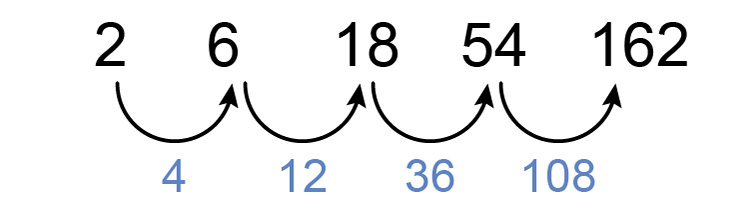No it's not

Is it quadratic - a consistent difference between differences?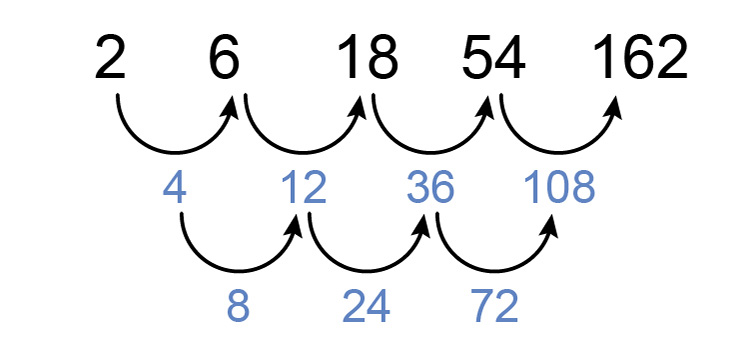No it's not

Is it a multiple (or geometric sequence)?

2,  6,  18,  54,  162

Divide each term by the previous term

6/2=3,  18/6=3,  54/18=3,  162/54=3

Yes, it is. It is a multiple of 3.

So the next two numbers are:

162times3=486

and the next would be

486times3=1,458

Example 2

What are the next two numbers in the following sequence?

-2,  4,  -8,  16,  -32,  64

Is it arithmetic - a consistent difference between numbers?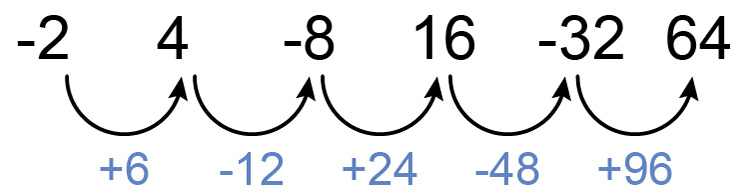No it's not

Is it a quadratic - a consistent difference between differences?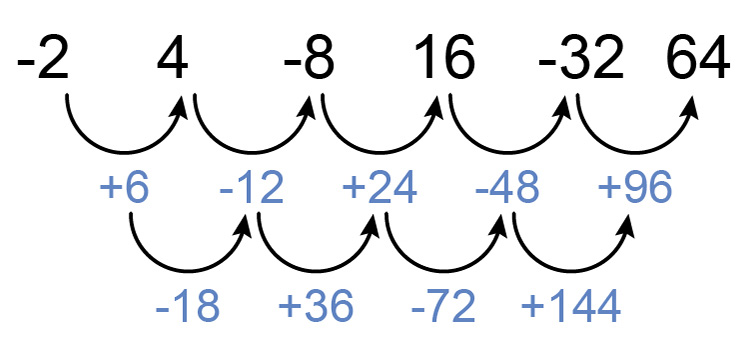No it's not

Is it a multiple (or geometric sequence)?

-2,  4,  -8,  16,  -32,  64

Divide each term by the previous term

4/-2=-2,  -8/4=-2,  16/-8=-2,  -32/16=-2,  64/-32=-2

Yes, it is. It is a multiple of -2.

So the next two numbers are:

64times-2=-128

and the next would be

-128times-2=256

Answer the next two numbers are -128  and 256

Example 3

What are the next two numbers in the following sequence?

40,  20,  10,  5,  2.5

Is it arithmetic - a consistent difference between numbers?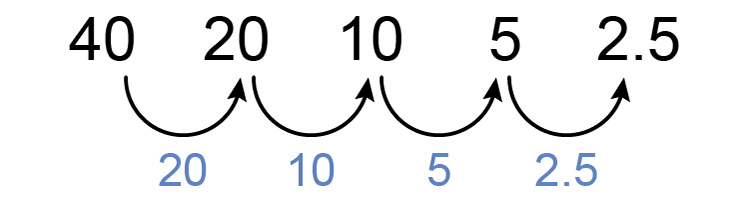No it's not

Is it quadratic - a consistent difference between differences?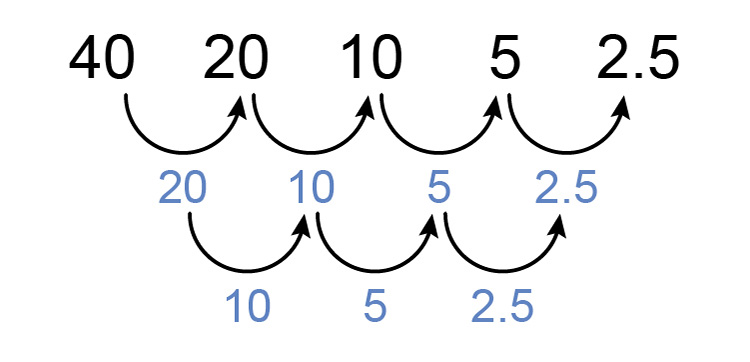No it's not

Is it a multiple (or geometric sequence)?

40,  20,  10,  5,  2.5

Divide each term by the previous term

20/40=0.5,  10/20=0.5,  5/10=0.5,  2.5/5=0.5

Yes, it is. It is a multiple of 0.5.

So the next two numbers are:

2.5times0.5=1.25

and the next would be

1.25times0.5=0.625

Answer the next two numbers are 1.25  and 0.625

Example 4

What are the next two numbers in the following sequence?

3,  3sqrt8,  24,  24sqrt8

This is a trick because the only way to tackle this is to:

1. Try and keep the first two sequences going, which is
3  and 3sqrt8
2. Then you have to recognise that
8=sqrt8timessqrt8

So this sequence could be rewritten

 3 =3 3sqrt8 =3sqrt8 24 = 3timessqrt8timessqrt8  (i.e. 3times8=24) =3sqrt8sqrt8 24sqrt8 = (3timessqrt8timessqrt8)timessqrt8 =3sqrt8sqrt8sqrt8

So the sequence can be rewritten as:

3,  3sqrt8,  3sqrt8sqrt8,  3sqrt8sqrt8sqrt8

Now you can clearly see that dividing each term by the previous term gives

(3sqrt8)/3=sqrt8,     (3sqrt8sqrt8)/(3sqrt8)=sqrt8,     (3sqrt8sqrt8sqrt8)/(3sqrt8sqrt8)=sqrt8

So this is a multiple of sqrt8

The next two numbers in the sequence are

3sqrt8sqrt8sqrt8timessqrt8=3times8times8=192

and the next is

3sqrt8sqrt8sqrt8sqrt8timessqrt8=3times8times8timessqrt8=192sqrt8

Answer next two numbers are 192  and 192sqrt8